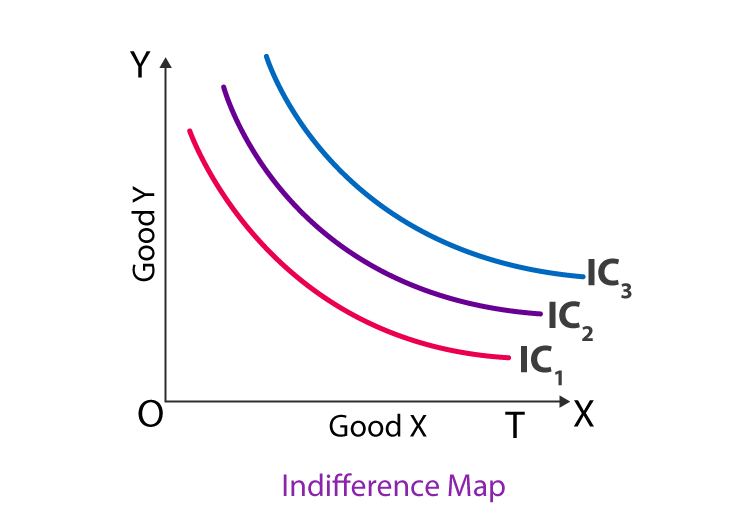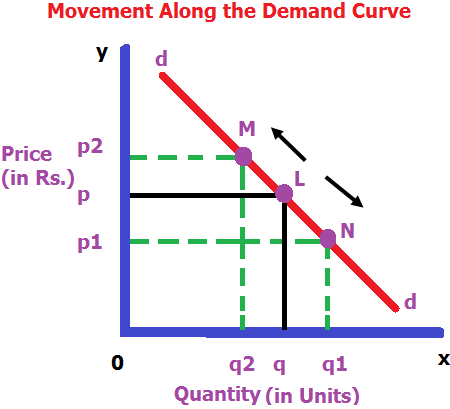# Indifference Curve

## What is Indifference Curve?

An indifference curve is a graphical representation of a combined products that gives similar kind of satisfaction to a consumer thereby making them indifferent.Every point on the indifference curve shows that an individual or a consumer is indifferent between the two products as it gives him the same kind of utility.

## Indifference Curve Analysis

The indifference curve analysis work on a simple graph having two-dimensional. Each individual axis indicates a single type of economic goods. If the graph is on the curve or line, then it means that the consumer has no preference for any goods, because all the good has the same level of satisfaction or utility to the consumer. For instance, a child might be indifferent while having a toy, two comic book, four toy trucks and a single comic book.

### Indifference Map

The Indifference Map refers to a set of Indifference Curves that reflects an understanding and gives an entire view of a consumer’s choices. The below diagram shows an indifference map with three indifference curves.Here, we understand that all three products resting in the indifferent curve give him the same satisfaction. However, his preference for those combined products can be arranged in the order of preference.

## Following are the features of indifference curve

(a) INDIFFERENCE CURVE ALWAYS SLOPES DOWNWARDS FROM LEFT TO RIGHT
• An indifference curve has a negative slope, i.e. it slopes downward from left to right.
• Reason: If a consumer decides to have one more unit of a commodity
• (say apples), quantity of another good (say oranges) must fall so that the total satisfaction (utility) remains same.

(a) INDIFFERENCE CURVE IS ALWAYS CONVEX TO THE ORIGIN
• IC is strictly Convex to origin i.e. MRSxy is always diminishing
• Reason: Due to the law of diminishing marginal utility a consumer is always willing to sacrifice lesser units of a commodity for every additional unit of another good.
(c) HIGHER INDIFFERENCE CURVE REPRESENTS

HIGHER LEVEL OF SATISFACTION

• Higher indifference curve represents larger bundles of goods i.e. bundles which contain more of both or more of at least one.
• It is assumed that consumer’s preferences are monotonic i.e. he always prefers larger bundle as it gives him higher satisfaction.• In the diagram, IC1 and IC2 are the two indifference curves. IC2 is the higher indifference curve than IC1.
• Combination ‘L’ contains more of both goods ‘X’ and Y than combination ‘M’ on IC1. Hence IC2 curve gives more satisfaction

### Lets Practise

1. The farther the Indifference curve from the origin, then:
1. Lower is the satisfaction level
2. Higher is the satisfaction level
3. Same satisfaction level will be obtained
4. None of the above

Answer: Higher is the satisfaction level

2. What is the shape of IC, when MRS between good X and Y is diminishing?
1. Straight line
2. Concave to the origin
3. Convex to the origin
4. None of these